Courses
Courses for Kids
Free study material
Free LIVE classes
More

# NCERT Solutions for Class 7 Maths Chapter 12 Algebraic Expressions In Hindi

## NCERT Solutions for Class 7 Maths Chapter 12 Algebraic Expressions In Hindi PDF Download

Last updated date: 25th Mar 2023
Total views: 351.9k
Views today: 10.28k

Download the Class 7 Maths NCERT Solutions in Hindi medium and English medium as well offered by the leading e-learning platform Vedantu. If you are a student of Class 7, you have reached the right platform. The NCERT Solutions for Class 7 Maths in Hindi provided by us are designed in a simple, straightforward language, which are easy to memorise. You will also be able to download the PDF file for NCERT Solutions for Class 7 Maths in Hindi from our website at absolutely free of cost.

NCERT, which stands for The National Council of Educational Research and Training, is responsible for designing and publishing textbooks for all the classes and subjects. NCERT textbooks covered all the topics and are applicable to the Central Board of Secondary Education (CBSE) and various state boards.

We, at Vedantu, offer free NCERT Solutions in English medium and Hindi medium for all the classes as well. Created by subject matter experts, these NCERT Solutions in Hindi are very helpful to the students of all classes.

## NCERT Solutions for Class 7 Maths Chapter 12 Algebraic Expressions In Hindi

प्रश्नावली 12.1

1. निम्नलिखित स्थितियों में, चरों, अचरों और अंकगणितीय संक्रियाओं का प्रयोग करते हुए, बीजीय व्यंजकप्राप्त कीजिए:

1.  संख्य y में से z को घटाना।

2.  संख्याओं x और yके योग का आधा।

3.  संख्या z  को स्वंय उस से गुणा किया जाता है।

4.  संख्याओं p और q के गुणनफल का एक-चौथाई।

5.  दोनों संख्याओं x और y के वर्गों को जोड़ा जाता है।

6.  संख्याओं m और n के गुणनफल के तीन गुने में संख्या 5 जोड़ना।

7.  10 में से संख्याओं  y और z गुणनफल को घटाना।

8.  संख्याओं a और b के गुणनफल में से उनके योग को घटाना।

उत्तर: $i)y-z$

$ii)\frac{x+y}{2}$

$iii){{z}^{2}}$

$iv)\frac{pq}{4},$

$v){{x}^{2}}+{{y}^{2}}$

$vi)3mn+5$

$vii)10-yz$

$viii)ab-\left( a+b \right)$

2. (i) निम्नलिखित व्यंजकों मेंप दों और उनके गुणनखंडों को छाँटिए।पदों और उनके गुणनखंडों को पेड़ आरेखों द्वारा भी दर्शाइए।

$\left( \mathbf{a} \right)~\mathbf{x}-\mathbf{3}$

उत्तर: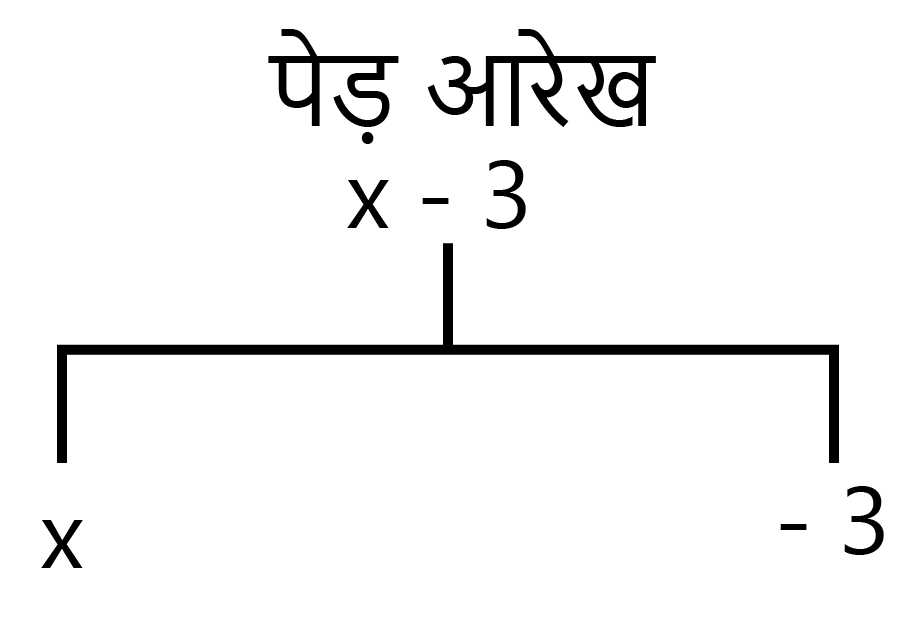$\left( \mathbf{b} \right)~\mathbf{1}+\mathbf{x}+{{\mathbf{x}}^{\mathbf{2}}}$

उत्तर: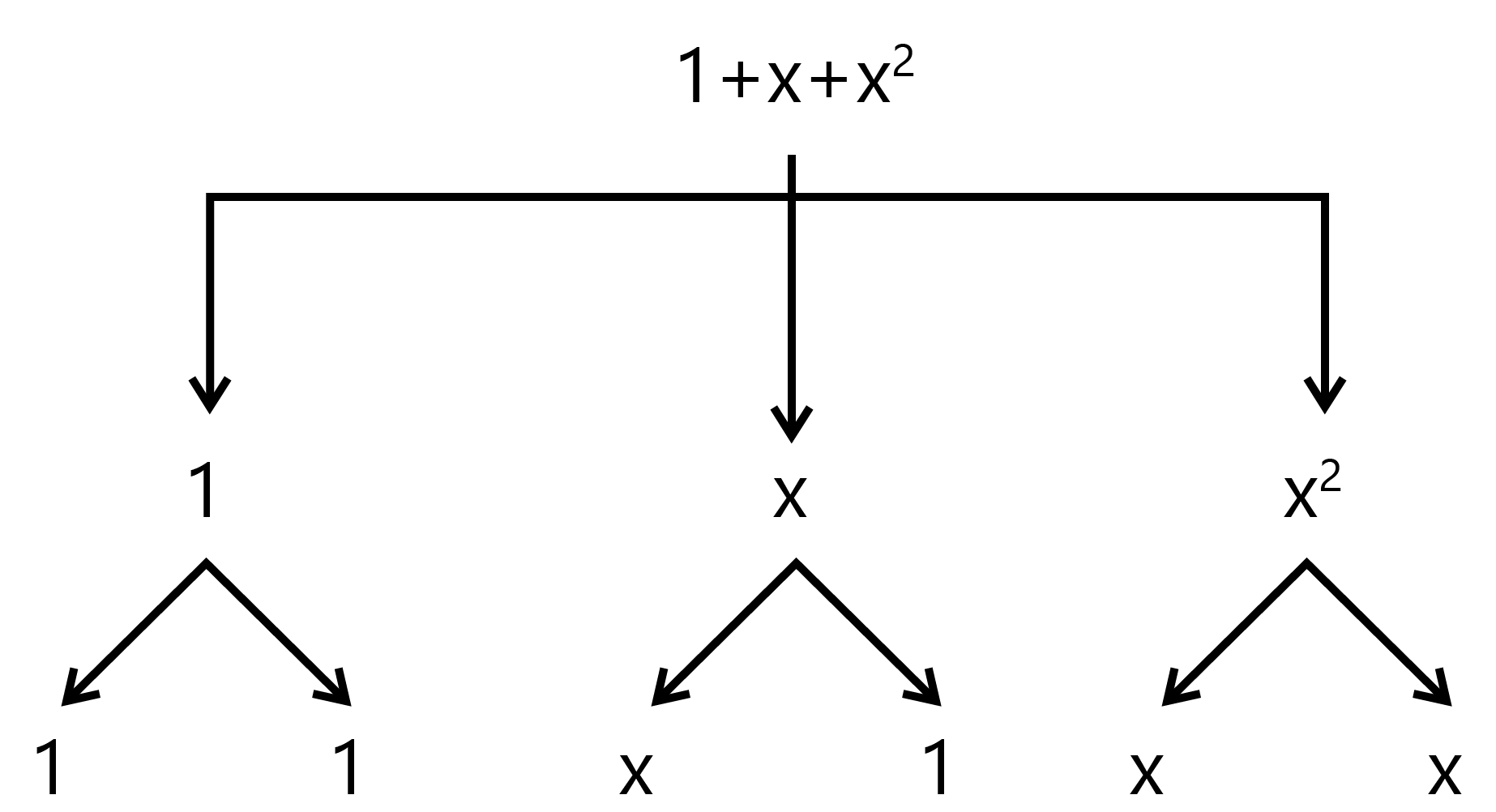$\left( \mathbf{c} \right)~\mathbf{y}-{{\mathbf{y}}^{\mathbf{3}}}$

उत्तर: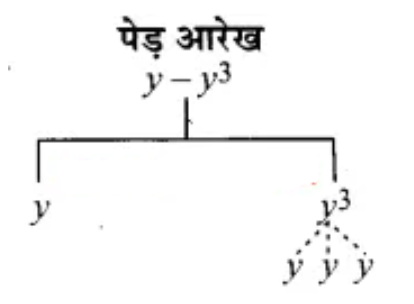$\left( \mathbf{d} \right)~\mathbf{5x}{{\mathbf{y}}^{\mathbf{2}}}+\mathbf{7}{{\mathbf{x}}^{\mathbf{2}}}\mathbf{y}$

उत्तर: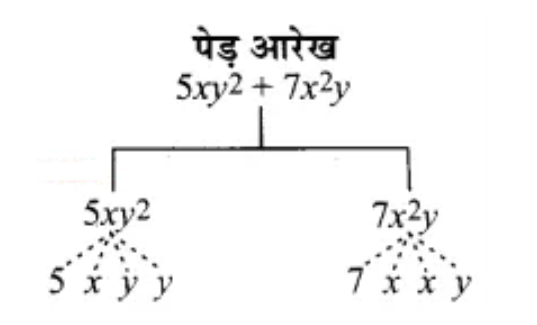$\left( \mathbf{e} \right)~-\mathbf{ab}+\mathbf{2}{{\mathbf{b}}^{\mathbf{2}}}-\mathbf{3}{{\mathbf{a}}^{\mathbf{2}}}$

उत्तर: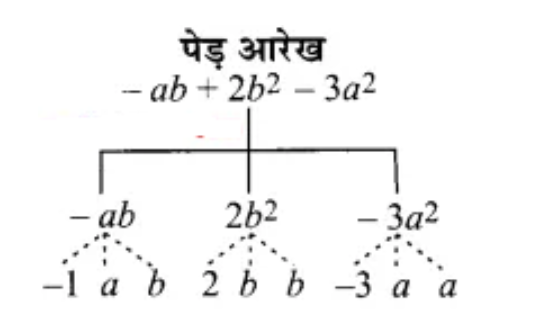(ii)नीचे दिए गए व्यंजकों में, पदों और उनके गुणनखंड को छाँटिए।

$\left( \mathbf{a} \right)~-\mathbf{4x}+\mathbf{5}$

उत्तर: पद x और गुणनखंड$-4$

$\left( \mathbf{b} \right)~-\mathbf{4x}+\mathbf{5y}$

उत्तर: पद x और गुणनखंड$-4$, पद y और गुणनखंड 5

$\left( \mathbf{c} \right)~\mathbf{5y}+\mathbf{3}{{\mathbf{y}}^{\mathbf{2}}}$

उत्तर: पद y और गुणनखंड 5, पद ${{y}^{2}}$ और गुणनखंड$3$

$\left( \mathbf{d} \right)~\mathbf{xy}+\mathbf{2}{{\mathbf{x}}^{\mathbf{2}}}{{\mathbf{y}}^{\mathbf{2}}}$

उत्तर: पद $xy$ और गुणनखंड 1, पद ${{x}^{2}}{{y}^{2}}$ और गुणनखंड 2

$\left( \mathbf{e} \right)~\mathbf{pq}+\mathbf{q}$

उत्तर: पद $pq$और गुणनखंड 1, पद q और गुणनखंड 1

$\left( \mathbf{f} \right)~\mathbf{1}.\mathbf{2ab}-\mathbf{2}.\mathbf{4b}+\mathbf{3}.\mathbf{6a}$

उत्तर: पद ab और गुणनखंड1,2 पद b और गुणनखंड 2,4 पद a और गुणनखंड 3,6

$\left( \mathbf{g} \right)~\frac{\mathbf{3}}{\mathbf{4}}\mathbf{x}+\frac{\mathbf{1}}{\mathbf{4}}$

उत्तर: पद x और गुणनखंड $\frac{3}{4}$

$\left( \mathbf{h} \right)~\mathbf{1}.\mathbf{2}{{\mathbf{p}}^{\mathbf{2}}}+\mathbf{0}.\mathbf{2}{{\mathbf{q}}^{\mathbf{2}}}$

उत्तर: पद ${{p}^{2}}$ और गुणनखंड 1,2 पद ${{q}^{2}}$ औरगुणनखंड $0.2$

3. निम्नलिखित व्यंजकों में पदों के संख्यात्मक गुणांकों, जो अचर न हों, पहचान कीजिए।

$\left( \mathbf{i} \right)~\mathbf{5}-\mathbf{3}{{\mathbf{t}}^{\mathbf{2}}}$

उत्तर: पद ${{t}^{2}}$ और गुणनखंड$-3$

$\left( \mathbf{ii} \right)~\mathbf{1}+\mathbf{t}+{{\mathbf{t}}^{\mathbf{2}}}+{{\mathbf{t}}^{\mathbf{3}}}$

उत्तर: पद $t$ और गुणनखंड $1,$पद ${{t}^{2}}$ और गुणनखंड $1,$पद ${{t}^{3}}$ और गुणनखंड$1,$

$\left( \mathbf{iii} \right)~\mathbf{x}+\mathbf{2xy}+\mathbf{3y}$

उत्तर: पद $x$और गुणनखंड$1,$, पद $xy$ और गुणनखंड$2$, पद $y$ और गुणनखंड$3$

$\left( \mathbf{iv} \right)~\mathbf{100m}+\mathbf{1000n}$

उत्तर: पद $m$और गुणनखंड$100$, पद $n$ और गुणनखंड$1000$

$\left( \mathbf{v} \right)~-{{\mathbf{p}}^{\mathbf{2}}}{{\mathbf{q}}^{\mathbf{2}}}+\mathbf{7pq}$

उत्तर: पद ${{p}^{2}}{{q}^{2}}$ और गुणनखंड$-1$, पद $pq$ और गुणनखंड$7$

$\left( \mathbf{vi} \right)~\mathbf{1}.\mathbf{2a}+\mathbf{0}.\mathbf{8b}$

उत्तर: पद a और गुणनखंड 1.2, पद  b और गुणनखंड 0.8

$\left( \mathbf{vii} \right)~\mathbf{3}.\mathbf{14}{{\mathbf{r}}^{\mathbf{2}}}$

उत्तर: पद ${{r}^{2}}~$और गुणनखंड 3.14

$\left( \mathbf{viii} \right)~\mathbf{2}\left( \mathbf{l}+\mathbf{b} \right)$

उत्तर: पद l और गुणनखंड 2, पद  b और गुणनखंड 2

$\left( \mathbf{ix} \right)~\mathbf{0}.\mathbf{1y}+\mathbf{0}.\mathbf{01}{{\mathbf{y}}^{\mathbf{2}}}$

उत्तर: पद y और गुणनखंड 0.1,, पद ${{y}^{2}}$ और गुणनखंड 0.01

4.

1. वे पद पहचानिए जिनमें $x$ है और फिर इनमें $x$ का गुणांक लिखिए।

$\left( \mathbf{i} \right)~{{\mathbf{y}}^{\mathbf{2}}}\mathbf{x}+\mathbf{y}$

उत्तर: ${{y}^{2}}$

$\left( \mathbf{ii} \right)~\mathbf{13}{{\mathbf{y}}^{\mathbf{2}}}-\mathbf{8yx}$

उत्तर: $8y$

$\left( \mathbf{iii} \right)~\mathbf{x}+\mathbf{y}+\mathbf{2}$

उत्तर: $1$

$\left( \mathbf{iv} \right)~\mathbf{5}+\mathbf{z}+\mathbf{zx}$

उत्तर: $z$

$\left( \mathbf{v} \right)~\mathbf{1}+\mathbf{x}+\mathbf{xy}$

उत्तर: $1$और $y$

$\left( \mathbf{vi} \right)~\mathbf{12x}{{\mathbf{y}}^{\mathbf{2}}}+\mathbf{25}$

उत्तर: $12x$

$\left( \mathbf{vii} \right)~\mathbf{7}+\mathbf{x}{{\mathbf{y}}^{\mathbf{2}}}$

उत्तर: ${{y}^{2}}$

1. वे पद पहचानिए,  जिसमें ${{y}^{2}}$ और फिर इनमें ${{y}^{2}}$ का गुणांक लिखिए।

$\left( \mathbf{i} \right)~\mathbf{8}-\mathbf{x}{{\mathbf{y}}^{\mathbf{2}}}$

उत्तर: $x$

$\left( \mathbf{ii} \right)~\mathbf{5}{{\mathbf{y}}^{\mathbf{2}}}+\mathbf{7x}$

उत्तर: $5$

$\left( \mathbf{iii} \right)~\mathbf{2}{{\mathbf{x}}^{\mathbf{2}}}\mathbf{y}-\mathbf{15x}{{\mathbf{y}}^{\mathbf{2}}}+\mathbf{7}{{\mathbf{y}}^{\mathbf{2}}}$

उत्तर: $15x$ और$7$

5. निम्नलिखित व्यंजकों को एक पदी,  द्विपद और त्रिपद के रूप में वर्गीकृत कीजिए:

i) $~\mathbf{7y}-\mathbf{7z} \\$

ii)$~~{{\mathbf{y}}^{\mathbf{2}}}~~~~ \\$

iii)$~~~\mathbf{x}+\mathbf{y}-\mathbf{xy} \\$

iv)$~\mathbf{100}~ \\$

v)$~~\mathbf{ab}-\mathbf{a}-\mathbf{b} \\$

vi)$~~\mathbf{5}-\mathbf{3t} \\$

vii)$\ \mathbf{4}{{\mathbf{p}}^{\mathbf{2}}}\mathbf{q}-\mathbf{4}{{\mathbf{q}}^{\mathbf{2}}}\mathbf{p} \\$

viii)$\ \mathbf{7mn} \\$

ix)$~{{\mathbf{z}}^{\mathbf{2}}}-\mathbf{3z}+\mathbf{8} \\$

x)$~~{{\mathbf{a}}^{\mathbf{2}}}+{{\mathbf{b}}^{\mathbf{2}}} \\$

xi)$\ {{\mathbf{z}}^{\mathbf{2}}}+\mathbf{z}~~ \\$

xii)$\ \mathbf{1}+\mathbf{x}+{{\mathbf{x}}^{\mathbf{2}}} \\$

उत्तर:  एकपदी$(ii),\text{ }\left( iv \right),\text{ }\left( viii \right)$

द्विपद$(i),\text{ }\left( vi \right),\text{ }\left( vii \right),\text{ }\left( x \right),\text{ }\left( xi \right)$

त्रिपद$(iii),\text{ }\left( v \right),\text{ }\left( ix \right),\text{ }\left( xii \right)$

6. बताइए कि दिए हुए पदों के युग्म समान पदों के हैं या असमान पदों के हैं:

$\mathbf{I}.~\mathbf{1},\mathbf{100}~~~~$

$\mathbf{II}.~-\mathbf{7x},~\frac{\mathbf{5}}{\mathbf{2}}\mathbf{x}$

$\mathbf{III}.~~-\mathbf{29x},~-\mathbf{29y}$

$\mathbf{IV}.~~\mathbf{14xy},~\mathbf{42yx}$

$\mathbf{V}.~~\mathbf{4}{{\mathbf{m}}^{\mathbf{2}}}\mathbf{p},~\mathbf{4}{{\mathbf{p}}^{\mathbf{2}}}\mathbf{m}$

$\mathbf{VI}.~\mathbf{12xz},~\mathbf{12}{{\mathbf{x}}^{\mathbf{2}}}{{\mathbf{z}}^{\mathbf{2}}}$

उत्तर:  समान  पद$(i),\text{ }\left( ii \right),\text{ }\left( iv \right)$

असमान पद$(iii),\text{ }\left( v \right),\text{ }\left( vi \right)$

7. निम्नलिखित में समान पदों को छाँटिए:

$\left( \mathbf{a} \right)~-\mathbf{x}{{\mathbf{y}}^{\mathbf{2}}}-,~-\mathbf{4y}{{\mathbf{x}}^{\mathbf{2}}},~\mathbf{8}{{\mathbf{x}}^{\mathbf{2}}},~\mathbf{2x}{{\mathbf{y}}^{\mathbf{2}}},~\mathbf{7y},~-\mathbf{11}{{\mathbf{x}}^{\mathbf{2}}},~-\mathbf{100x},~-\mathbf{11yx},~\mathbf{20}{{\mathbf{x}}^{\mathbf{2}}}\mathbf{y},~\mathbf{6}{{\mathbf{x}}^{\mathbf{2}}},~\mathbf{y},~\mathbf{2xy},~\mathbf{3x}$

उत्तर: $\left( x{{y}^{2}},~2x{{y}^{2}} \right),\text{ }\left( 8{{x}^{2}}11{{x}^{2}} \right),\text{ }\left( -100x,~3x \right)$

$\left( b \right)~10pq,~7p~,~8q,~-{{p}^{2}}{{q}^{2}},~-7qp,~-100q,~-23,~12{{q}^{2}}{{p}^{2}},~-5{{p}^{2}},41,~2405p,~78qp,~13{{p}^{2}}q,~q{{p}^{2}},~701{{p}^{2}}$

उत्तर: $~\left( 10pq-7qp,~78qp \right),\text{ }\left( 7p,~2405p \right),\text{ }\left( -{{p}^{2}}{{q}^{2}},~12{{q}^{2}}{{p}^{2}} \right),\left( -\text{ }23,\text{ }41 \right)$

### प्रश्नावली 12.2

1. समान पदों को सन्योजित (मिला) कर सरल कीजिए:

1. $\left( \mathbf{a} \right)~\mathbf{21b}-\mathbf{32}+\mathbf{7b}-\mathbf{20b}$

उत्तर: $21b+7b-20b-32$

$=28b-20b-32$

$=8b-32$

1. $\left( \mathbf{b} \right)~-{{\mathbf{z}}^{\mathbf{2}}}+\mathbf{13}{{\mathbf{z}}^{\mathbf{2}}}-\mathbf{5z}+\mathbf{7}{{\mathbf{z}}^{\mathbf{3}}}-\mathbf{15z}$

उत्तर: $7{{z}^{3}}-{{z}^{2}}+13{{z}^{2}}-5z-15z$

$=7{{z}^{3}}+12{{z}^{2}}-20z$

1. $\left( \mathbf{c} \right)~\mathbf{p}-\left( \mathbf{p}-\mathbf{q} \right)-\mathbf{q}-\left( \mathbf{q}-\mathbf{p} \right)$

उत्तर: $p-p+q-q-q+p$

$=p-p+p+q-q-q$

$=p-q$

1. $\left( \mathbf{d} \right)~\mathbf{3a}-\mathbf{2b}-\mathbf{ab}-\left( \mathbf{a}-\mathbf{b}+\mathbf{ab} \right)+\mathbf{3ab}+\mathbf{b}-\mathbf{a}$

उत्तर: $3a-2b-ab-a+b-ab+3ab+b-a$

$=3a-a-a-2b+b+b-ab-ab+3ab$

$=a-ab$

1. $\left( \mathbf{e} \right)~\mathbf{5}{{\mathbf{x}}^{\mathbf{2}}}\mathbf{y}-\mathbf{5}{{\mathbf{x}}^{\mathbf{2}}}+\mathbf{3y}{{\mathbf{x}}^{\mathbf{2}}}-\mathbf{3}{{\mathbf{y}}^{\mathbf{2}}}+{{\mathbf{x}}^{\mathbf{2}}}-{{\mathbf{y}}^{\mathbf{2}}}+\mathbf{8x}{{\mathbf{y}}^{\mathbf{2}}}-\mathbf{3}{{\mathbf{y}}^{\mathbf{2}}}$

उत्तर:

$5{{x}^{2}}y+3{{x}^{2}}y-5{{x}^{2}}+{{x}^{2}}-3{{y}^{2}}-{{y}^{2}}-3{{y}^{2}}+8x{{y}^{2}} \\$

$=\ 8{{x}^{2}}y-4{{x}^{2}}-7{{y}^{2}}+8x{{y}^{2}} \\$

1. $\left( \mathbf{f} \right)~\left( \mathbf{3}{{\mathbf{y}}^{\mathbf{2}}}+\mathbf{5y}-\mathbf{4} \right)-(\mathbf{8y}-{{\mathbf{y}}^{\mathbf{2}}}-\mathbf{4})$

उत्तर: $3{{y}^{2}}+5y-4-8y+{{y}^{2}}+4$

$=3{{y}^{2}}+{{y}^{2}}+5y-8y-4+4$

$=4{{y}^{2}}-3y$

2. जोड़िए:

1. $\left( \mathbf{a} \right)~\mathbf{3mn},-\mathbf{5mn},\mathbf{8mn},-\mathbf{4mn}$

उत्तर:

$3mn-5mn+8mn-4mn \\$

$= \ 3mn+8mn-5mn-4mn \\$

$= \ 11mn-9mn=2mn \\$

1. $\left( \mathbf{b} \right)~\mathbf{t}-\mathbf{8tz},\mathbf{3tz}-\mathbf{z},\mathbf{z}-\mathbf{t}$

उत्तर:

$~t-8t.z+3t.z-z+z-t \\$

$t-t-z+z-8t.z+3t.z \\$

$= \ - \ 8t.z+3t.z=-5t.z \\$

1. $\left( \mathbf{c} \right)~-\mathbf{5mn}+\mathbf{5},\mathbf{12mn}+\mathbf{2},\mathbf{9mn}-\mathbf{8},-\mathbf{2mn}-\mathbf{3}$

उत्तर:

$- \ 5mn+5+12mn+2+9mn-8-2mn-3 \\$

$= \ - \ 5mn+12mn+9mn-2mn+5+2-8-3 \\$

$= \ 14mn+7-11=14mn-4 \\$

1. $\left( \mathbf{d} \right)~\mathbf{a}+\mathbf{b}-\mathbf{3},\mathbf{b}-\mathbf{a}+\mathbf{3},\mathbf{a}-\mathbf{b}+\mathbf{3}$+3,a-b+3

उत्तर: $a \ + \ b \ - \ 3 \ + \ b \ - \ a \ + \ 3 \ + \ a \ - \ b \ + \ 3 \\$

$= \ a\ - \ a \ + \ a \ + \ b \ + \ b \ - \ b \ - \ 3 \ + \ 3 \ + \ 3 \\$

$= \ a \ + \ b \ + \ 3 \\$

1. $\left( \mathbf{e} \right)~\mathbf{14x}+\mathbf{10y}-\mathbf{12xy}-\mathbf{13},\mathbf{18}-\mathbf{7x}-\mathbf{10}+\mathbf{8xy},\mathbf{4xy}$

उत्तर:  $14x \ + \ 10y \ - \ 12xy \ - \ 13 \ + \ 18 \ - \ 7x \ - \ 10 \ + \ 8xy \ + \ 4xy \\$

$= \ 14x \ - \ 7x \ + \ 10y \ - \ 12xy \ + \ 8xy \ + \ 4xy \ - \ 13 \ + \ 18 \ - \10 \\$

$=7x-2y-23+18=7x-2y-5 \\$

1. $\left( \mathbf{f} \right)~\mathbf{5m}-\mathbf{7n},\mathbf{3n}-\mathbf{4m}+\mathbf{2},\mathbf{2m}-\mathbf{3mn}-\mathbf{5}$

उत्तर:

$5m \ -\ 7n \ + \ 3n \ - \ 4m \ + \ 2 \ + \ 2m \ - \ 3mn \ - \ 5 \\$

$= \ 5m \ - \ 4m \ + \ 2m \ - \ 7n \ + \ 3n \ - \ 3mn \ + \ 2 \ - \ 5 \\$

=3m-4n-3mn-3  \\$1. $\left( \mathbf{g} \right)~\mathbf{4}{{\mathbf{x}}^{\mathbf{2}}}\mathbf{y},\mathbf{3x}{{\mathbf{y}}^{\mathbf{2}}},-\mathbf{5x}{{\mathbf{y}}^{\mathbf{2}}},\mathbf{5}{{\mathbf{x}}^{\mathbf{2}}}\mathbf{y}$ उत्तर:$   4{{x}^{2}}y+3x{{y}^{2}}-5x{{y}^{2}}+5{{x}^{2}}y  \\   = \ 4xy \ + \ {5x}^{2} y \ + \ 3xy^2 \ - \ 5xy^2  \\

$= \ 9xy \ - \ 2x{{y}^{2}} \\$

1. $\left( \mathbf{h} \right)~\mathbf{3}{{\mathbf{p}}^{\mathbf{2}}}{{\mathbf{q}}^{\mathbf{2}}}-\mathbf{4pq}+\mathbf{5},-\mathbf{10}{{\mathbf{p}}^{\mathbf{2}}}{{\mathbf{q}}^{\mathbf{2}}},\mathbf{15}+\mathbf{9pq}+\mathbf{7}{{\mathbf{p}}^{\mathbf{2}}}{{\mathbf{q}}^{\mathbf{2}}}$

उत्तर:

$3{{p}^{2}}{{q}^{2}}-4pq+5-10{{p}^{2}}{{q}^{2}}+15+9pq+7{{p}^{2}}{{q}^{2}} \\$

$=3{{p}^{2}}{{q}^{2}}-10{{p}^{2}}{{q}^{2}}+7{{p}^{2}}{{q}^{2}}-4pq+9pq+5+15 \\$

$=10{{p}^{2}}{{q}^{2}}-10{{p}^{2}}{{q}^{2}}+9pq-4pq+20 \\$

$=5pq+20 \\$

1. $\left( \mathbf{i} \right)~\mathbf{ab}-\mathbf{4a},\mathbf{4b}-\mathbf{ab},\mathbf{4a}-\mathbf{4b}$

उत्तर: $ab-4a+4b-ab+4a-4b \\$

$=ab-ab-4a+4a+4b-4b \\$

$=0 \\$

1. $\left( \mathbf{j} \right)~{{\mathbf{x}}^{\mathbf{2}}}-{{\mathbf{y}}^{\mathbf{2}}}-\mathbf{1},{{\mathbf{y}}^{\mathbf{2}}}-\mathbf{1}-{{\mathbf{x}}^{\mathbf{2}}},\mathbf{1}-{{\mathbf{x}}^{\mathbf{2}}}-{{\mathbf{y}}^{\mathbf{2}}}$

उत्तर: ${{x}^{2}}-{{y}^{2}}-1+{{y}^{2}}-1-{{x}^{2}}+1-{{x}^{2}}-{{y}^{2}} \\$

$= \ {{x}^{2}}-{{x}^{2}}-{{x}^{2}}-{{y}^{2}}+{{y}^{2}}-{{y}^{2}}-1-1+1 \\$

$= \ -{{x}^{2}}-{{y}^{2}}-1 \\$

3. घटाइए:

1. ($\left( \mathbf{a} \right)~{{\mathbf{y}}^{\mathbf{2}}}$में से $~\mathbf{5}-{{\mathbf{y}}^{\mathbf{2}}}$

उत्तर:

${{y}^{2}}-\left( 5-{{y}^{2}} \right) \\$

$={{y}^{2}}-5+{{y}^{2}} \\$

$=2{{y}^{2}}-5 \\$

1. $\left( \mathbf{b} \right)~-\mathbf{12xy}~$में से $\mathbf{6xy}$

उत्तर: $-12xy-6xy=-18xy$

1. $\left( \mathbf{c} \right)~\left( \mathbf{a}+\mathbf{b} \right)$में से $\left( \mathbf{a}-\mathbf{b} \right)$

उत्तर:

$a+b-\left( a-b \right) \\$

$=a+b-a+b=2b \\$

1. $\left( \mathbf{d} \right)~\mathbf{b}\left( \mathbf{5}-\mathbf{a} \right)~$में से $\mathbf{a}\left( \mathbf{b}-\mathbf{5} \right)$

उत्तर:

$~b\left( 5-a \right)-a\left( b-5 \right) \\$

$=\left( 5b-ab \right)-\left( ab-5a \right) \\$

$=5b-ab-\left( ab-5a \right) \\$

$=5b-ab-ab+5a \\$

$=5a+5b-2ab \\$

1. $\left( \mathbf{e} \right)~\mathbf{4}{{\mathbf{m}}^{\mathbf{2}}}-\mathbf{3mn}+\mathbf{8}$में से $-{{\mathbf{m}}^{\mathbf{2}}}+\mathbf{5mn}$

उत्तर:

$~4{{m}^{2}}-3mn+8-\left( -m2+5mn \right) \\$

$=4{{m}^{2}}-3mn+8+{{m}^{2}}-5mn \\$

$=4{{m}^{2}}+{{m}^{2}}-3mn-5mn+88 \\$

$=5{{m}^{2}}-8mn+8 \\$

1. $\left( \mathbf{f} \right)~\mathbf{5x}-\mathbf{10}~$में से $-{{\mathbf{x}}^{\mathbf{2}}}+\mathbf{10x}-\mathbf{5}$

उत्तर:

$5x-10-\left( -x2+10x-5 \right) \\$

$=5x-10+x2-10x+5 \\$

$={{x}^{2}}+5x-10x-10+5 \\$

$={{x}^{2}}-5x-5 \\$

1. $\mathbf{g})~\mathbf{3ab}-\mathbf{2}{{\mathbf{a}}^{\mathbf{2}}}-\mathbf{2}{{\mathbf{b}}^{\mathbf{2}}}$से $\mathbf{5}{{\mathbf{a}}^{\mathbf{2}}}-\mathbf{7ab}+\mathbf{5}{{\mathbf{b}}^{\mathbf{2}}}$

उत्तर:

$3ab-2{{a}^{2}}-2{{b}^{2}}-\left( 5{{a}^{2}}-7ab+5{{b}^{2}} \right) \\$

$=3ab-2{{a}^{2}}-2{{b}^{2}}-5{{a}^{2}}+7ab-5{{b}^{2}} \\$

$=3ab+7ab-2{{a}^{2}}-5{{a}^{2}}-2{{b}^{2}}-5{{b}^{2}} \\$

$=10ab-7{{a}^{2}}-7{{b}^{2}} \\$

1. $\left( \mathbf{h} \right)~\mathbf{5}{{\mathbf{p}}^{\mathbf{2}}}+\mathbf{3}{{\mathbf{q}}^{\mathbf{2}}}-\mathbf{pq}~$में से $\mathbf{4pq}-\mathbf{5}{{\mathbf{q}}^{\mathbf{2}}}-\mathbf{3}{{\mathbf{p}}^{\mathbf{2}}}$

उत्तर:

$5{{p}^{2}}+3{{q}^{2}}-pq-\left( 4pq-5{{q}^{2}}-3{{p}^{2}} \right) \\$

$=5{{p}^{2}}+3{{q}^{2}}-pq-4pq+5{{q}^{2}}+3{{p}^{2}} \\$

$=5{{p}^{2}}+3{{p}^{2}}+3{{q}^{2}}+5{{q}^{2}}-pq-4pq \\$

$=8{{p}^{2}}+8{{q}^{2}}-5pq \\$

4. $\left( \mathbf{a} \right)~\mathbf{2}{{\mathbf{x}}^{\mathbf{2}}}+\mathbf{3xy}~$प्राप्त करने के लिए ${{\mathbf{x}}^{\mathbf{2}}}+\mathbf{xy}+{{\mathbf{y}}^{\mathbf{2}}}~$में क्या जोड़ना चाहिए?

उत्तर: $2{{x}^{2}}+3xy-\left( {{x}^{2}}+xy+{{y}^{2}} \right) \\$

$=2{{x}^{2}}+3xy-{{x}^{2}}-xy-{{y}^{2}} \\$

$=2{{x}^{2}}-{{x}^{2}}+3xy-xy-{{y}^{2}} \\$

$={{x}^{2}}+2xy-{{y}^{2}} \\$

4. $\left( \mathbf{b} \right)~-\mathbf{3a}+\mathbf{7b}+\mathbf{16}~$प्राप्त करने के लिए, $\mathbf{2a}+\mathbf{8b}+\mathbf{10}~$में से क्या घटाना चाहिए?

उत्तर:  $2a+8b+10-\left( -3a+7b+16 \right) \\$

$=2a+8b+10+3a-7b-16 \\$

$=2a+3a+8b-7b+10-16 \\$

$=5a+b-6 \\$

5.$~-{{\mathbf{x}}^{\mathbf{2}}}-{{\mathbf{y}}^{\mathbf{2}}}+\mathbf{6xy}+\mathbf{20}~$प्राप्त करने के लिए, $\mathbf{3}{{\mathbf{x}}^{\mathbf{2}}}-\mathbf{4}{{\mathbf{y}}^{\mathbf{2}}}+\mathbf{5xy}+\mathbf{20}~$में क्या निकाल लेना चाहिए?

उत्तर:

$3{{x}^{2}}-4{{y}^{2}}+5xy+20-\left( -{{x}^{2}}-{{y}^{2}}+6xy+20 \right) \\$

$=3{{x}^{2}}-4{{y}^{2}}+5xy+20+{{x}^{2}}+{{y}^{2}}-6xy-20 \\$

$=3{{x}^{2}}+{{x}^{2}}-4{{y}^{2}}+{{y}^{2}}+5xy-6xy+20-20 \\$

$=4{{x}^{2}}-3{{y}^{2}}-xy \\$

6(a). $\left( \mathbf{a} \right)~\mathbf{3x}-\mathbf{y}+\mathbf{11}~$और $-\mathbf{y}-\mathbf{11}~$के योग में से $\mathbf{3x}-\mathbf{y}-\mathbf{11}~$को घटाइए।

उत्तर: $3x-y+11-y-11-\left( 3x-y-11 \right) \\$

$=3x-y+11-y-11-3x+y+11 \\$

$=3x-3x-y-y+y+11-11+11 \\$

$=y+11 \\$

6(b). $\left( \mathbf{b} \right)~\mathbf{4}+\mathbf{3}{{\mathbf{x}}^{\mathbf{4}}}+\mathbf{3x}~$और $\mathbf{5}-\mathbf{4x}+\mathbf{2}{{\mathbf{x}}^{\mathbf{2}}}~$के योग में से $\mathbf{3}{{\mathbf{x}}^{\mathbf{2}}}-\mathbf{5x}~$और $-{{\mathbf{x}}^{\mathbf{2}}}+\mathbf{2x}+\mathbf{5}~$के योग को घटाइए।

उत्तर: $\left( 4+3x+5-4x+2{{x}^{2}} \right)-\left( 3{{x}^{2}}-5x-{{x}^{2}}+2x+5 \right) \\$

$=\left( 2{{x}^{2}}+3x-4x+4+5 \right)-\left( 3{{x}^{2}}-{{x}^{2}}-5x+2x+5 \right) \\$

$=\left( 2{{x}^{2}}-x+9 \right)-\left( 2{{x}^{2}}-3x+5 \right) \\$

$=2{{x}^{2}}-x+9-2{{x}^{2}}+3x-5 \\$

$=2{{x}^{2}}-2{{x}^{2}}-x+3x+9-5 \\$

$=2x+4 \\$

### प्रश्नावली 12.3

1. यदि $\mathbf{m}=\mathbf{2}~$है, तो निम्नलिखित के मान ज्ञात कीजिए:

1. $\mathbf{m}-\mathbf{2}$

उत्तर:

$m-2 \\$

$= \ 2-2 \\$

$= \ 0 \\$

1. $\mathbf{3m}-\mathbf{5}$

उत्तर:

$3m \ - \ 5 \\$

$= \ 3\times 2-5 \\$

$= \ 6 - 5 \\$

$= \ 1 \\$

1. $\mathbf{9}-\mathbf{5m}$

उत्तर:

$9 \ - \ 5m \\$

$= \ 9 \ - \ 5 \times 2 \\$

$= \ 9 \ - \ 10 \\$

$= \ -1 \\$

1. $\mathbf{3}{{\mathbf{m}}^{\mathbf{2}}}-\mathbf{2m}-\mathbf{7}$

उत्तर:

$\ 3{{m}^{2}} \ - 2m -7 \\$

$= \ 3 \times {{2}^{2}}-2\times 2 \ - \ 7 \\$

$= \ 3 \times 4 \ - \ 4 \ - \ 7 \\$

$= \ 12 \ - \ 4 \ - \ 7 \\$

$= \ 1 \\$

1. $\frac{\mathbf{5m}}{2}-\mathbf{4}$

उत्तर:

$\frac{5m}{2} \ - \ 4 \\$

$= \ \frac{5\times 2}{2} \ - \ 4 \\$

$= \ 5 \ - \ 4 \\$

$= \ - \ 1 \\$

2. यदि $\mathbf{p}=-\mathbf{2}$, तो निम्नलिखित के मान ज्ञात कीजिए:

1. $\mathbf{4p}+\mathbf{7}$

उत्तर:

$4 \ \left( -2 \right) \ + \ 7 \\$

$= \ - \ 8 \ + \ 7 \\$

$= \ - \ 1 \\$

1. ${-3p^2} + 4p + 7$

उत्तर:

$- \ 3 \ \times {{\left( -2 \right)}^{2}}+4\times \left( -2 \right) \ + \ 7 \\$

$= \ - \ 3 \ \times 4 \ - \ 8 \ + \ 7 \\$

$= \ - \ 12 \ - \ 8 \ + \ 7 \\$

$= \ - \ 13 \\$

1. $\mathbf{2}{{\mathbf{p}}^{\mathbf{3}}}-\mathbf{3}{{\mathbf{p}}^{\mathbf{2}}}+\mathbf{4p}+\mathbf{7}$

उत्तर:

$- \ 2 \ \times \left( -2 \right) \ 3 \ - \ 3 \ {{\left( -2 \right)}^{2}} \ + \ 4 \ \left( -2 \right) \ + \ 7 \\$

$= \ - \ 2 \ \times \left( -8 \right) \ - \ 3 \times 4 \ - \ 8 \ + \ 7 \\$

$= \ 16 \ - \ 12 \ - \ 1 \\$

$= \ 3 \\$

3. निम्नलिखित व्यंजकों के मान ज्ञात कीजिए, जब $\mathbf{x}=-\mathbf{1}~$है:

1. ($\mathbf{2x}-\mathbf{7}$

उत्तर:

$2 \ \left( -1 \right) \ - \ 7 \\$

$= \ - \ 2 \ - \ 7 \\$

$= \ - \ 9 \\$

1. $\mathbf{x}+\mathbf{2}$

उत्तर:

$- \ \left( -1 \right) \ + \ 2 \\$

$= \ 1 \ + \ 2 \\$

$= \ 3 \\$

1. ${{\mathbf{x}}^{\mathbf{2}}}+\mathbf{2x}+\mathbf{1}$

उत्तर:

${{\left( -1 \right)}^{2}} \ + \ 2 \left( -1 \right) \ + \ 1 \\$

$= \ 1 \ - \ 2 \ + \ 1 \\$

$= \ 0 \\$

1. $\mathbf{2}{{\mathbf{x}}^{\mathbf{2}}}-\mathbf{2x}-\mathbf{2}$

उत्तर:

$2{{\left( -1 \right)}^{2}}-2\left( -1 \right)-2$

$= \ 2 \ + \ 2 \ - \ 2 \\$

$= \ 2 \\$

4. यदि $\mathbf{a}=\mathbf{2}~$और $\mathbf{b}=-\mathbf{2}~$है,  तो निम्नलिखित के मान ज्ञात कीजिए:

1. ${{\mathbf{a}}^{\mathbf{2}}}+{{\mathbf{b}}^{\mathbf{2}}}$

उत्तर:

$\ ~22 \ + \ {{\left( -2 \right)}^{2}} \\$

$= \ 4 \ + \ 4 \\$

$= \ 8 \\$

1. ${{\mathbf{a}}^{\mathbf{2}}}+\mathbf{ab}+{{\mathbf{b}}^{\mathbf{2}}}$

उत्तर:

${{2}^{2}} \ + \ 2\left( -2 \right) \ + \ {{\left( -2 \right)}^{2}} \\$

$= \ 4 \ - \ 4 \ + \ 4 \\$

$= \ 4 \\$

1. ${{\mathbf{a}}^{\mathbf{2}}}-{{\mathbf{b}}^{\mathbf{2}}}$

उत्तर:

${{2}^{2}}-{{\left( -2 \right)}^{2}} \\$

$=4-4 \\$

$=0 \\$

5. जब $\mathbf{a}=\mathbf{0}~$और $\mathbf{b}=-\mathbf{1}~$है, तो दिए हुए व्यंजकों के मान ज्ञात कीजिए:

1. $\mathbf{2a}+\mathbf{ab}$

उत्तर:

$2\times 0+0\left( -1 \right) \\$

$=0+0 \\$

$=0 \\$

1. $\mathbf{2}{{\mathbf{a}}^{\mathbf{2}}}+{{\mathbf{b}}^{\mathbf{2}}}+\mathbf{1}$

उत्तर:

$2\times {{0}^{2}}+{{\left( -1 \right)}^{2}}+1 \\$

$=1+1 \\$

$=2 \\$

1. $\mathbf{2}{{\mathbf{a}}^{\mathbf{2}}}\mathbf{b}+\mathbf{2}{{\mathbf{b}}^{\mathbf{2}}}+\mathbf{ab}$

उत्तर:

$2\times {{0}^{2}}\times \left( -1 \right)+2{{\left( -1 \right)}^{2}}+0\left( -1 \right) \\$

$=2$

1. ${{\mathbf{a}}^{\mathbf{2}}}+\mathbf{ab}+\mathbf{2}$

उत्तर:

${{0}^{2}}+0\left( -1 \right)+2 \\$

$=2 \\$

6. इन व्यंजकों को सरल कीजिए तथा इनके मान ज्ञात कीजिए, जब $x$ का मान$\mathbf{2}$है:

1. $\mathbf{x}+\mathbf{7}+\mathbf{4}\left( \mathbf{x}-\mathbf{5} \right)$

उत्तर:

$2+7+4\left( 2-5 \right) \\$

$=9+4\left( -3 \right) \\$

$=9-12 \\$

$=-3 \\$

1. $\mathbf{3}\left( \mathbf{x}+\mathbf{2} \right)+\mathbf{5x}-\mathbf{7}$

उत्तर:

$3\left( 2+2 \right)+5\times 2-7$

$=3\times 4+10-7 \\$

$=12+10-7 \\$

$=15 \\$

1. $\mathbf{6x}+\mathbf{5}\left( \mathbf{x}-\mathbf{2} \right)$

उत्तर: $6\times 2+5\left( 2-2 \right) \\$

$=12 \\$

1. $\mathbf{4}\left( \mathbf{2x}-\mathbf{1} \right)+\mathbf{3x}+\mathbf{11}$

उत्तर: $\left( 2\times 2-1 \right)+3\times 2+11 \\$

$=4\left( 4-1 \right)+6+11 \\$

$=4\times 3+17 \\$

$=12+17 \\$

$=29 \\$

7. इन व्यंजकों को सरल कीजिए तथा इन के मान ज्ञात कीजिए, जब $\mathbf{x}=\mathbf{3},~\mathbf{a}=-\mathbf{1}~$और $\mathbf{b}=-\mathbf{2}~$है:

1. $\mathbf{3x}-\mathbf{5}-\mathbf{x}+\mathbf{9}$

उत्तर:  $3\times 3-5-3-9 \\$

$=9-17 \\$

$=-8 \\$

1. $\mathbf{2}-\mathbf{8x}+\mathbf{4x}+\mathbf{4}$

उत्तर: $2-8\times 3+4\times 3+4 \\$

$=2-24+12+4 \\$

$=-6 \\$

1. $\mathbf{3a}+\mathbf{5}-\mathbf{8a}+\mathbf{1}$

उत्तर:

$3\left( -1 \right)+5-8\left( -1 \right)+1 \\$

$=-3+5-8+1 \\$

$=-11+6 \\$

$=-5 \\$

1. $\mathbf{10}-\mathbf{3b}-\mathbf{4}-\mathbf{5b}$

उत्तर: $10-3\left( -2 \right)-4-5\left( -2 \right)\grave{\ } \\$

$=10+6-4+10 \\$

$=22 \\$

1. $\left( \mathbf{e} \right)~\mathbf{2a}-\mathbf{2b}-\mathbf{4}-\mathbf{5}+\mathbf{a}$

उत्तर: $2\left( -1 \right)-2\left( -2 \right)-4-5-1 \\$

$=-2+4-10-8 \\$

$=-16 \\$

8.

1. यदि $\mathbf{z}=\mathbf{10}~$है, तो ${{\mathbf{z}}^{\mathbf{3}}}-\mathbf{3}\left( \mathbf{z}-\mathbf{10} \right)~$का मान ज्ञात कीजिए।

उत्तर: $103-3\left( 10-10 \right) \\$

$=1000-0 \\$

$=1000 \\$

1. यदि $\mathbf{p}=-\mathbf{10}~$है, तो ${{\mathbf{p}}^{\mathbf{2}}}-\mathbf{2p}-\mathbf{100}~$का मान ज्ञात कीजिए।

उत्तर: ${{\left( -10 \right)}^{2}}-2\left( -10 \right)-100)$

$=100+20-100 \\$

$=20 \\$

9. यदि $\mathbf{x}=\mathbf{0}~$पर $\mathbf{2}{{\mathbf{x}}^{\mathbf{2}}}+\mathbf{x}-\mathbf{a}~$का मान$\mathbf{5}$के बराबर है, तो $\mathbf{a}$ का मान क्या होना चाहिए?

उत्तर:  $2\times {{0}^{2}}+0-a \\$

$=5 \\$

या, $-a=5$

या, $a=-5$

10. व्यंजक$~\mathbf{2}\left( {{\mathbf{a}}^{\mathbf{2}}}+\mathbf{ab} \right)+\mathbf{3}-\mathbf{ab}~$को सरल कीजिए और इसका मान ज्ञात कीजिए, जब $\mathbf{a}=\mathbf{5}~$और $\mathbf{b}=-\mathbf{3}~$है।

उत्तर: $~2\left( {{5}^{2}}+5\left( -3 \right) \right)+3-5\left( -3 \right) \\$

$=2\left( 25-15 \right)+3+15 \\$

$=2\times 10+18 \\$

$=20+18 \\$

$=38 \\$

### NCERT Solutions for Class 7 Maths Chapter 12 Algebraic Expressions In Hindi

Chapter-wise NCERT Solutions are provided everywhere on the internet with an aim to help the students to gain a comprehensive understanding. Class 7 Maths Chapter 12 solution Hindi mediums are created by our in-house experts keeping the understanding ability of all types of candidates in mind. NCERT textbooks and solutions are built to give a strong foundation to every concept. These NCERT Solutions for Class 7 Maths Chapter 12 in Hindi ensure a smooth understanding of all the concepts including the advanced concepts covered in the textbook.

NCERT Solutions in Hindi medium have been created keeping those students in mind who are studying in a Hindi medium school. These NCERT Solutions for Class 7 Maths Algebraic Expressions in Hindi medium pdf download have innumerable benefits as these are created in simple and easy-to-understand language. The best feature of these solutions is a free download option. Students of Class 7 can download these solutions at any time as per their convenience for self-study purpose.

These solutions are nothing but a compilation of all the answers to the questions of the textbook exercises. The answers/ solutions are given in a stepwise format and very well researched by the subject matter experts who have relevant experience in this field. Relevant diagrams, graphs, illustrations are provided along with the answers wherever required. In nutshell, NCERT Solutions for Class 7 Maths in Hindi come really handy in exam preparation and quick revision as well prior to the final examinations.

## FAQs on NCERT Solutions for Class 7 Maths Chapter 12 Algebraic Expressions In Hindi

1. What are the main topics covered in NCERT Solutions for Class 7 Maths Chapter 12?

NCERT Solutions for Class 7 maths chapter 12 covers terms of expression, monomials, binomials, trinomials, like and unlike fractions, addition and subtraction of algebraic expressions, polynomials. It also helps you to find the value of an expression and how to use algebraic expressions. NCERT solutions include all the formulas and rules that are mentioned in the NCERT Book. This list of topics is prepared after examining the NCERT Book and keeping in mind the syllabus provided.

2. What are algebraic expressions in Class 7?

When an equation includes a constant, variable and an algebraic operation such as addition, subtraction, multiplication or subtraction, the equation can be defined as an algebraic expression. The algebraic expressions are further divided into five categories. If you want a further explanation or details about the five categories then you can go through NCERT Solutions for Class 7 maths on Vedantu’s website (vedantu.com). NCERT solutions will provide you with no excessive information and can also be downloaded free of cost.

3. How do you solve algebraic expressions in Grade 7?

The basic step is to solve the numericals in the equation with the help of algebraic operations as and when needed to isolate the variable. The next step is to solve the constant i.e. find its value. You can understand this process through NCERT solutions. It will provide you with a step by step guide on how to do the algebraic expression. You can also look up some educational videos explaining the same process too. All the study materials are available on the Vedantu app.

4. How do you multiply algebraic expressions in Class 7?

Multiplication of algebraic expression includes multiplication of equations including variables and constants. With a bracket in the equation, it is obvious to solve the bracket first. If the equation lacks brackets then it is given that first, you need to multiply or divide them then carry out addition or subtraction. You need to follow the order of BODMAS. Go through NCERT solutions to understand this concept with examples.

5. Why is Class 7 Maths Chapter 12 so difficult?

Some of you might find Chapter 12 ' Algebraic expression’ a tad difficult as compared to other chapters. The chapter consists of new terms and concepts such as linear questions with constants and variables, coefficients, monomials, polynomials etc. Do not get afraid of these fancy words. The concepts are easy to grasp. With continuous practice and effort, you will understand the terms and concepts. The formulas and rules are also easy to understand.